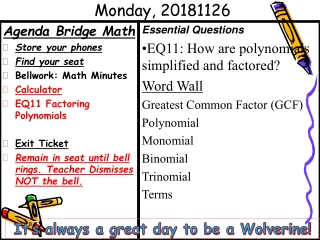DownloadDownload PresentationMonday, 20181126

# Monday, 20181126

Télécharger la présentation## Monday, 20181126

- - - - - - - - - - - - - - - - - - - - - - - - - - - E N D - - - - - - - - - - - - - - - - - - - - - - - - - - -
##### Presentation Transcript

1. Monday, 20181126 Essential Questions • EQ11: How are polynomials simplified and factored? Word Wall Greatest Common Factor (GCF) Polynomial Monomial Binomial Trinomial Terms Agenda Bridge Math • Store your phones • Find your seat • Bellwork: Math Minutes • Calculator • EQ11 Factoring Polynomials • Exit Ticket • Remain in seat until bell rings. Teacher Dismisses NOT the bell. It’s always a great day to be a Wolverine!

3. WW#2 Bridge Math Make-up from Tuesday-20181120 • WW#2 Bridge Math  join.quizizz.com

4. X-box Factoring standard quadratics (ax2+bx+c)

5. X- Box x2-6x-27 Product -27 3 -9 Sum -6 Factors (x-9) (x+3) Solution: x2-6x-27=

6. X-box Factoring • This is a guaranteed method for factoring standard quadratics (ax2+bx+c) —no guessing necessary! • We will learn how to factor quadratic equations using the x-box method • Background knowledge needed: • Basic x-solve problems • General form of a quadratic equation (ax2+bx+c) • Dividing a polynomial by a monomial using the box method

7. Students apply basic factoring techniques to second- and simple third-degree polynomials. These techniques include finding a common factor for all terms in a polynomial, recognizing the difference of two squares, and recognizing perfect squares of binomials. Objective: I can use the x-box method to factor non-prime trinomials.

8. -12 4 Examples Factor using the x-box method. 1. x2 + 4x – 12 a) b) x +6 x26x -2x -12 x 6 -2 -2 Solution: x2 + 4x – 12 = (x + 6)(x - 2)

9. Examples continued 2. x2 - 9x + 20 a) b) x -4 -4-5 20 -9 x2-4x -5x 20 x -5 Solution: x2 - 9x + 20 =(x - 4)(x - 5)

10. Think-Pair-Share • Based on the problems we’ve done, list the steps in the diamond/box factoring method so that someone else can do a problem using only your steps. Trying out the Steps • Trade papers with your partner and use their steps to factor the following problem: x2 +4x -32. • If you cannot complete the problem using only the steps written, put an arrow on the step where you stopped. Give your partner’s paper back to him. Stepping Up • Modify the steps you wrote to correct any incomplete or incorrect steps. Finish the problem based on your new steps and give the steps back to your partner. • Try using the steps again to factor: 4x2 +4x -3. • Edit your steps and factor: 3x2 + 11x – 20. • Formalize the steps as a class.

11. Formalize the steps as a class.Stepping Up Step 1: Write the problem. Step 2: Write c value on top, b value on bottom of X Step 3: Find factors of c, to multiply to c value, sum to b value Step 4: Write the a value in 4 square box, write the c value in 4 square box Step 5: Combine like terms. Step 6: Write the 2 binomial answer in form

12. Stop for Practice

13. Independent Practice Factor the standard quadratics (ax2+bx+c) on worksheets using the x-box method. Show all your work to receive credit– don’t forget to check by multiplying!

14. EXIT TICKET Please complete these individually. 1. Fill in the following X-solve problems. a. b. c. 2. Write the standard form of a quadratic equation.